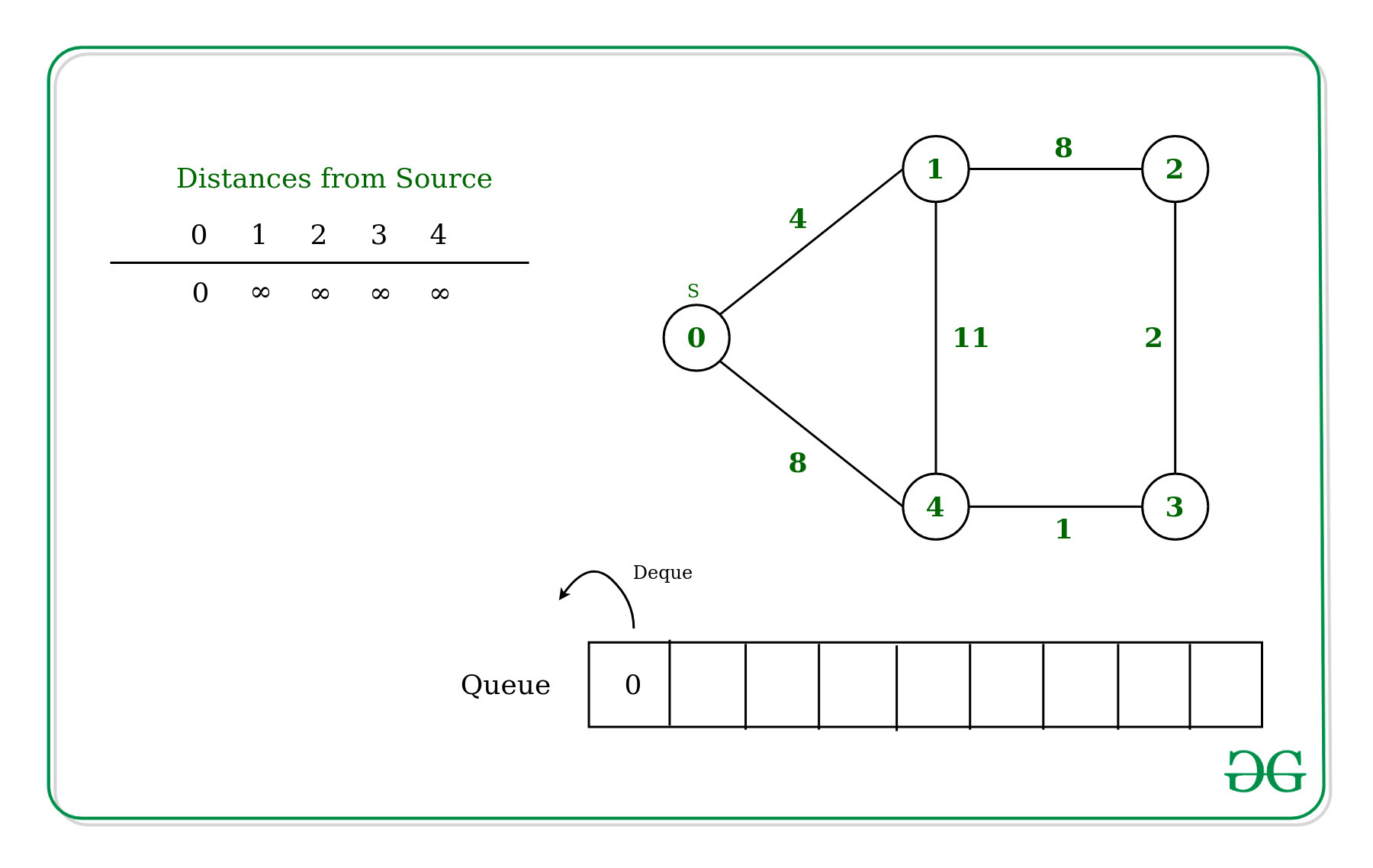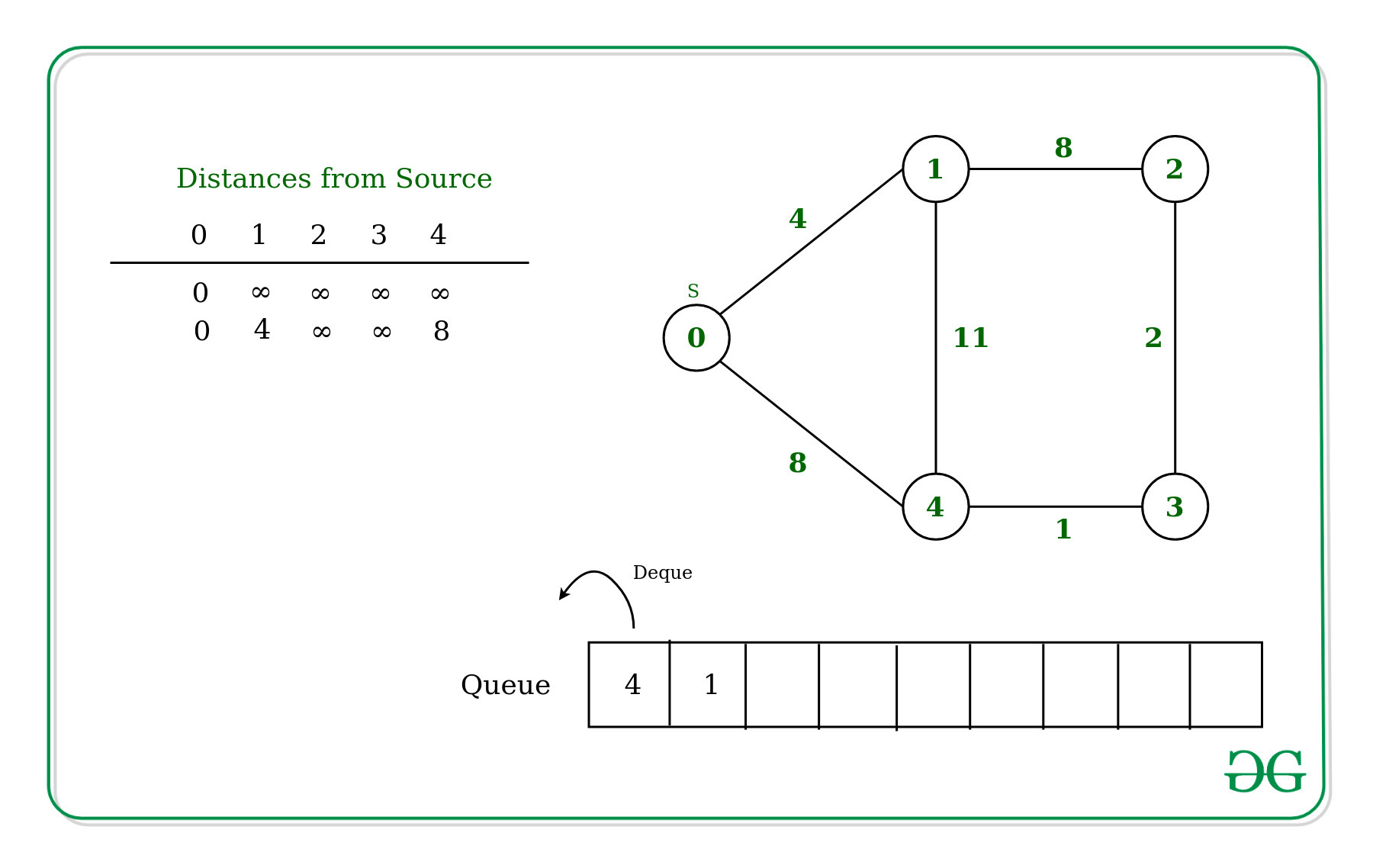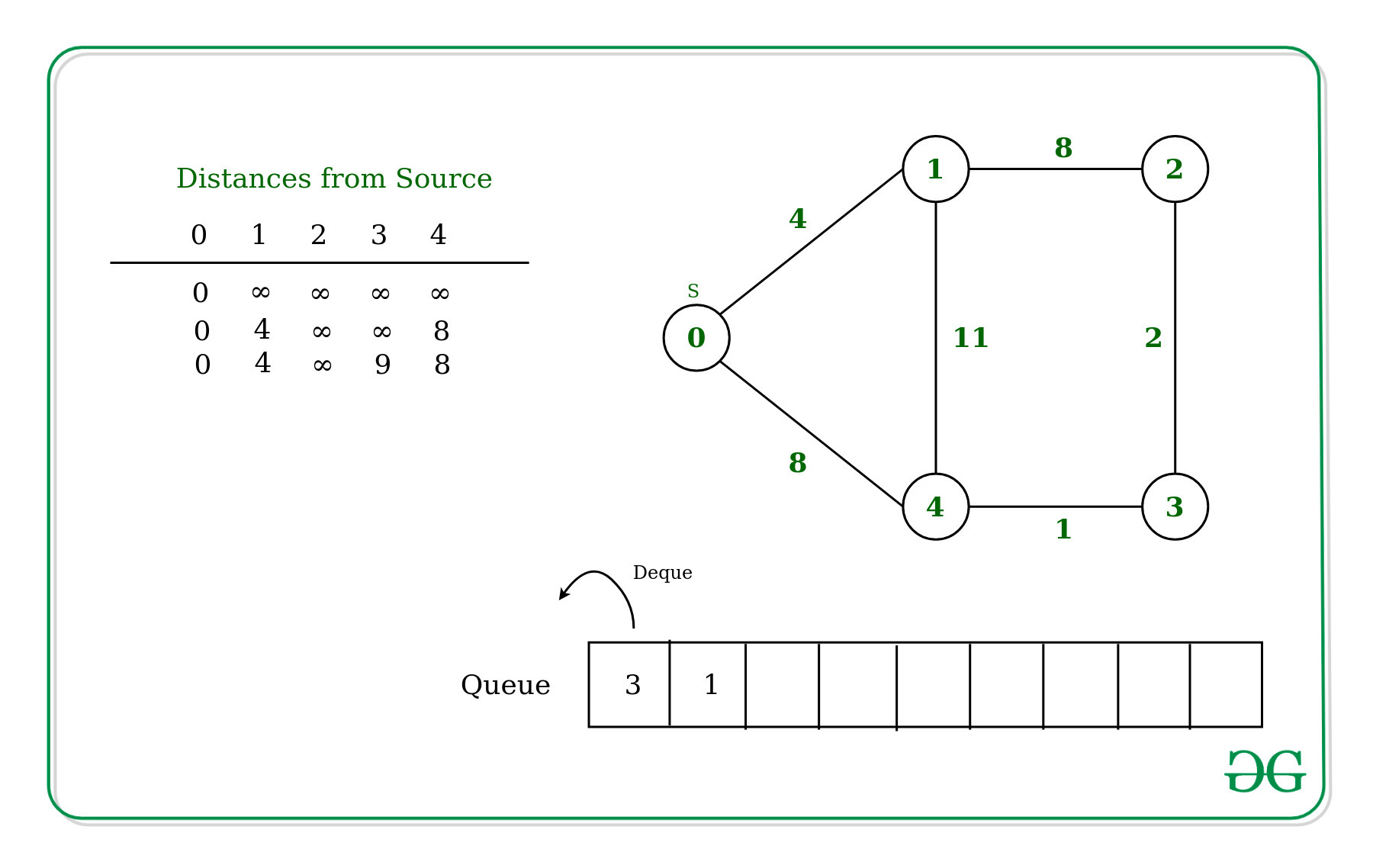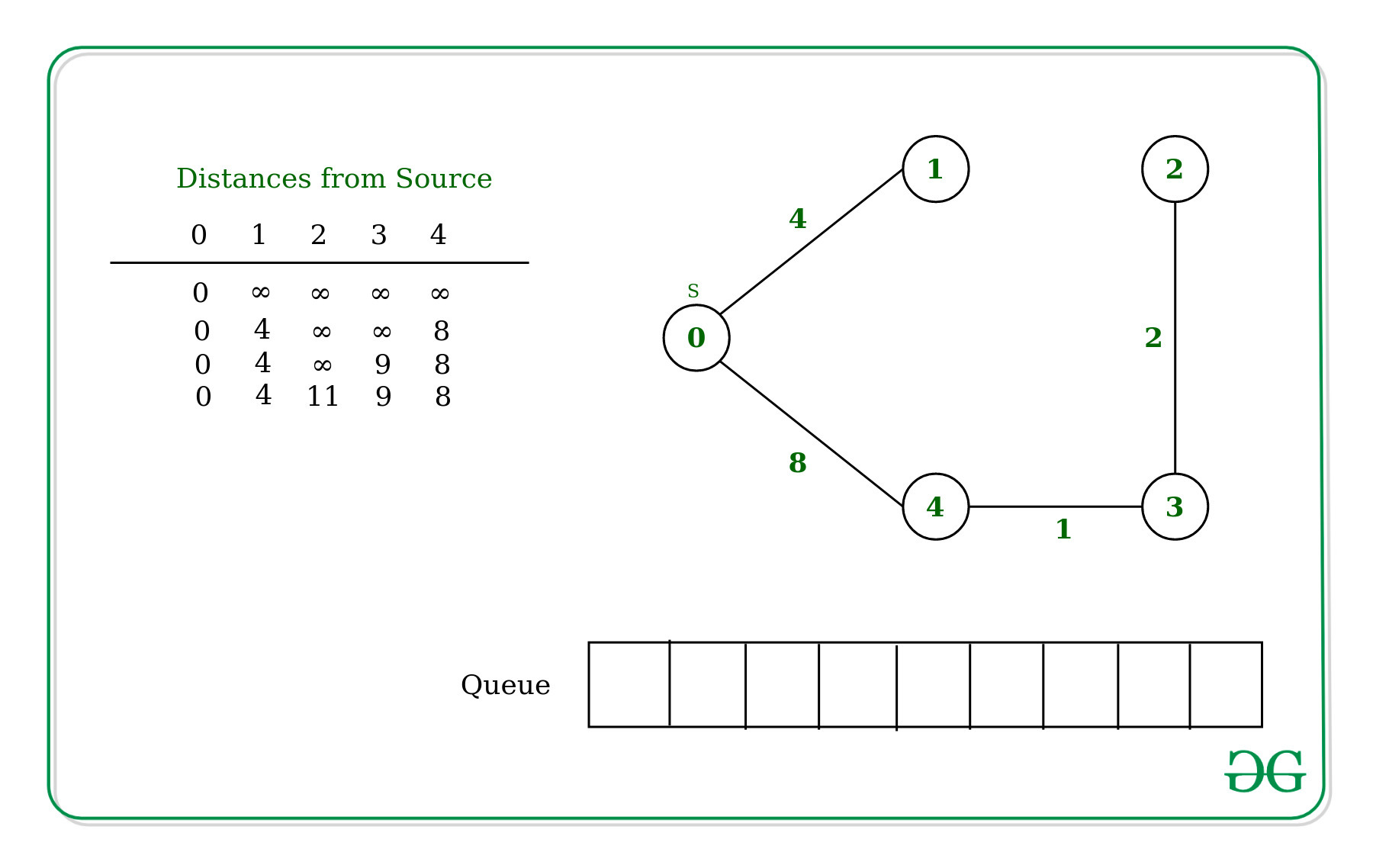D’Esopo-Pape Algorithm : Single Source Shortest Path

• Last Updated : 28 Dec, 2020

Given a graph and a source vertex src in a weighted undirected graph, find the shortest paths from src to all vertices in the given graph. The graph may contain negative weight edges.

For this problem, we have already discussed Dijkstra’s algorithm and Bellman-Ford Algorithm. But D’Esopo-Pape Algorithm performs quite well in most of the cases. However there are some cases in which it takes exponential time.

Algorithm:
Input: Adjacency List of the graph and source vertex src.
Output: Shortest distance to all vertices from src.
This Algorithm uses a Bi-directional queue that stores the vertices to be operated.

Following are the detailed steps of the algorithm.

1. Initialize distance of the vertices from source to infinite in an array.
2. Maintain a Queue which will store the vertices to be operated and also maintain a Boolean array for vertices which will be used to decide that the vertex is already present in the queue or not.
3. Append source vertex in the queue.
4. Start popping vertices from the queue until queue is empty, and perform following steps for each popped vertex(let U be the popped vertex) :
• Set the vertex U as not present in the queue.
• For each adjacent vertex V of U, check if its current minimum Distance[V] is greater than the distance through U
i.e. Distance[U] + weight of edge connecting U and V.
• If yes, update Distance[V] = Distance[U] + weight of edge connecting U and V.
Check If V is not present in the queue with the help of Boolean Array:
1. If V is entering the queue for the first time, append V at the back of the queue and set vertex V as present in the queue with the help of Boolean Array.
2. Else append in the front of the queue and set V vertex as present in the queue.
5. Return list Distance which will have shortest distance of every vertex from the source vertex.

For Example:
Initially, the Distance from source to itself will be 0 and for other vertices it will be infinite.Now for each Adjacent vertex of source that is 0 in this case are [1, 4] update the distance and mark the vertices as present in the with weight of 4 and 8 respectively.Now Deque the vertex 4 from the queue and following are the adjacent vertices are connected to the vertex 4 –

• Vertex 1 – As Vertex 1 have already visited and the weight to reach the vertex 1 is 4, whereas when move to vertex 1 via the edge 4 — 1 from source the total weight will be 11 which is greater than weight stored in the distance array.
• Vertex 3 – As Vertex 3 is not visited and also not present in the queue, So the distance is updated to 9 for vertex 3 and also enqueued into the queue at the front.Similarly Deque the vertex 3 from the Queue and update the values for adjacent vertex. Adjacent vertices of the vertex 3 is vertex 4 and vertex 2.

• Vertex 4 – As vertex 4 is already visited and the weight is already minimum So Distance is not updated.
• Vertex 2 – As Vertex 2 is not visited and also not present in the queue, So the distance is updated to 11 for vertex 3 and also enqueued into the queue at the front.Below is the implementation of the above approach.

C++

 // C++ implementation for // D'Esopo-Pape algorithm #include using namespace std; #define inf INT_MAX   vector desopo(vector> &graph) {       // Number of vertices in graph   int v = graph.size();     // Adjacency list of graph   map>> adj;   for(int i = 0; i < v; i++) {     for(int j = i + 1; j < v; j++)     {       if (graph[i][j] != 0)       {         adj[i].push_back({graph[i][j], j});         adj[j].push_back({graph[i][j], i});       }     }   }     // Queue to store unoperated vertices   deque q;     // Distance from source vertex   // distance =[float('inf')]*v   vector distance(v, inf);     // Status of vertex   vector is_in_queue(v, false);     // let 0 be the source vertex   int source = 0;   distance = 0;   q.push_back(source);   is_in_queue = true;     while (!q.empty())   {           // Pop from front of the queue     int u = q.front();     q.pop_front();     is_in_queue[u] = false;       // Scan adjacent vertices of u     for(auto e : adj[u])     {               // e <- [weight, vertex]       if (distance[e.second] >           distance[u] + e.first)       {         distance[e.second] = distance[u] + e.first;         if (!is_in_queue[e.second])         {                       // if e.second is entering           // first time in the queue           if (distance[e.second] == inf)                           // Append at back of queue             q.push_back(e.second);           else                           // Append at front of queue             q.push_front(e.second);                       is_in_queue[e.second] = true;         }       }     }   }   return distance; }   // Driver Code int main(int argc, char const *argv[]) {       // Adjacency matrix of graph   vector> graph = { { 0, 4, 0, 0, 8 },                                 { 0, 0, 8, 0, 11 },                                 { 0, 8, 0, 2, 0 },                                 { 0, 0, 2, 0, 1 },                                 { 8, 11, 0, 1, 0 } };   for(auto i : desopo(graph))   {     cout << i << " ";   }   return 0; }   // This code is contributed by sanjeev2552

Python3

 # Python3 implementation for # D'Esopo-Pape algorithm   from collections import defaultdict, deque   def desopo(graph):     # Number of vertices in graph     v = len(graph)           # Adjacency list of graph     adj = defaultdict(list)     for i in range(v):         for j in range(i + 1, v):             if graph[i][j] != 0:                 adj[i].append(                     [graph[i][j], j]                 )                 adj[j].append(                     [graph[i][j], i]                 )                 # Queue to store unoperated vertices     q = deque([])           # Distance from source vertex     distance =[float('inf')]*v           # Status of vertex     is_in_queue =[False]*v           # let 0 be the source vertex     source = 0     distance= 0     q.append(source)     is_in_queue= True           while q:         # Pop from front of the queue         u = q.popleft()         is_in_queue[u]= False               # scan adjacent vertices of u         for e in adj[u]:             # e <- [weight, vertex]             if distance[e] > distance[u]+e:                 distance[e]= distance[u]+e                 if is_in_queue[e]== False:                     # if e is entering                     # first time in the queue                     if distance[e]== float('inf'):                         # Append at back of queue                         q.append(e)                     else:                         # Append at front of queue                         q.appendleft(e)                     is_in_queue[e] = True     return distance     # Driver Code if __name__ == "__main__":     # Adjacency matrix of graph     graph = [[0, 4, 0, 0, 8],             [0, 0, 8, 0, 11],             [0, 8, 0, 2, 0],             [0, 0, 2, 0, 1],             [8, 11, 0, 1, 0]             ]     print(desopo(graph))

Output:

[0, 4, 11, 9, 8]

My Personal Notes arrow_drop_up
Recommended Articles
Page :